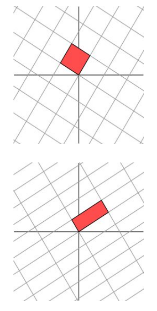# 1. 奇异值分解原理

## 1.1 特征值的数学含义# 2. 奇异值分解解线性方程

## 2.2 解齐次线性方程组($Ax=0$)

2.1的情况是让$||Ax-b||{2}$的值最小，但2.2由于$b=0$所以是让$||Ax||{2}$最小，由于$x=0$是方程的解，而我们一般不想要这个解，所以增加一个约束$||x||_2=1$，这样问题就变成了：